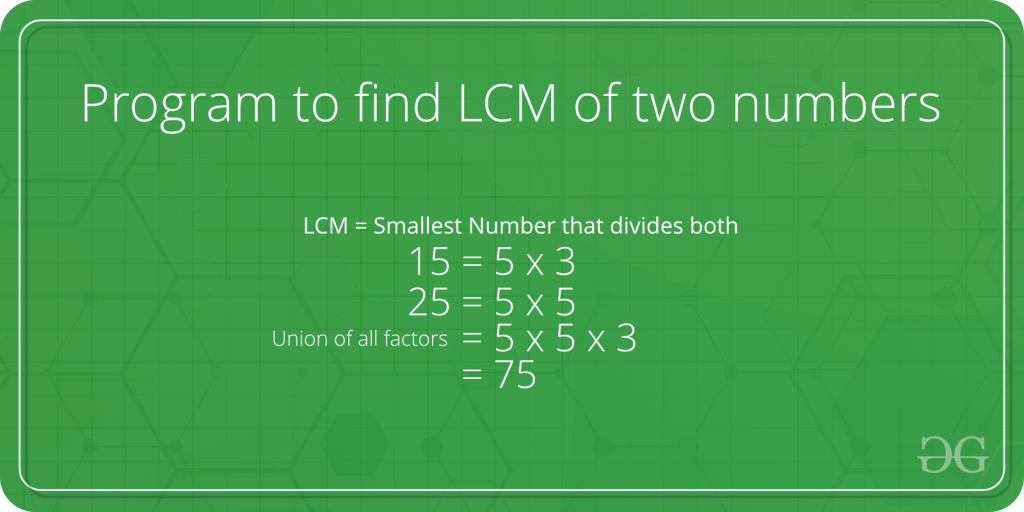Related Articles
Program to find LCM of two numbers
• Difficulty Level : Easy
• Last Updated : 18 Mar, 2021LCM (Least Common Multiple) of two numbers is the smallest number which can be divided by both numbers.

For example, LCM of 15 and 20 is 60, and LCM of 5 and 7 is 35.

A simple solution is to find all prime factors of both numbers, then find union of all factors present in both numbers. Finally, return the product of elements in union.

An efficient solution is based on the below formula for LCM of two numbers ‘a’ and ‘b’.

```   a x b = LCM(a, b) * GCD (a, b)

LCM(a, b) = (a x b) / GCD(a, b)```

We have discussed function to find GCD of two numbers. Using GCD, we can find LCM.

Below is the implementation of the above idea:

## C++

 `// C++ program to find LCM of two numbers``#include ``using` `namespace` `std;` `// Recursive function to return gcd of a and b``long` `long` `gcd(``long` `long` `int` `a, ``long` `long` `int` `b)``{``  ``if` `(b == 0)``    ``return` `a;``  ``return` `gcd(b, a % b);``}` `// Function to return LCM of two numbers``long` `long` `lcm(``int` `a, ``int` `b)``{``    ``return` `(a / gcd(a, b)) * b;``}`` ` `// Driver program to test above function``int` `main()``{``    ``int` `a = 15, b = 20;``    ``cout <<``"LCM of "` `<< a << ``" and "``         ``<< b << ``" is "` `<< lcm(a, b);``    ``return` `0;``}`

## C

 `// C program to find LCM of two numbers``#include ` `// Recursive function to return gcd of a and b``int` `gcd(``int` `a, ``int` `b)``{``    ``if` `(a == 0)``        ``return` `b;``    ``return` `gcd(b % a, a);``}` `// Function to return LCM of two numbers``int` `lcm(``int` `a, ``int` `b)``{``    ``return` `(a / gcd(a, b)) * b;``}` `// Driver program to test above function``int` `main()``{``    ``int` `a = 15, b = 20;``    ``printf``(``"LCM of %d and %d is %d "``, a, b, lcm(a, b));``    ``return` `0;``}`

## Java

 `// Java program to find LCM of two numbers.``class` `Test``{``    ``// Recursive method to return gcd of a and b``    ``static` `int` `gcd(``int` `a, ``int` `b)``    ``{``        ``if` `(a == ``0``)``            ``return` `b;``        ``return` `gcd(b % a, a);``    ``}``    ` `    ``// method to return LCM of two numbers``    ``static` `int` `lcm(``int` `a, ``int` `b)``    ``{``        ``return` `(a / gcd(a, b)) * b;``    ``}``    ` `    ``// Driver method``    ``public` `static` `void` `main(String[] args)``    ``{``        ``int` `a = ``15``, b = ``20``;``        ``System.out.println(``"LCM of "` `+ a +``                           ``" and "` `+ b +``                      ``" is "` `+ lcm(a, b));``    ``}``}`

## Python3

 `# Python program to find LCM of two numbers` `# Recursive function to return gcd of a and b``def` `gcd(a,b):``    ``if` `a ``=``=` `0``:``        ``return` `b``    ``return` `gcd(b ``%` `a, a)` `# Function to return LCM of two numbers``def` `lcm(a,b):``    ``return` `(a ``/` `gcd(a,b))``*` `b` `# Driver program to test above function``a ``=` `15``b ``=` `20``print``(``'LCM of'``, a, ``'and'``, b, ``'is'``, lcm(a, b))` `# This code is contributed by Danish Raza`

## C#

 `// C# program to find LCM``// of two numbers.``using` `System;``class` `GFG {``    ` `    ``// Recursive method to``    ``// return gcd of a and b``    ``static` `int` `gcd(``int` `a, ``int` `b)``    ``{``        ``if` `(a == 0)``            ``return` `b;``        ``return` `gcd(b % a, a);``    ``}``    ` `    ``// method to return``    ``// LCM of two numbers``    ``static` `int` `lcm(``int` `a, ``int` `b)``    ``{``        ``return` `(a / gcd(a, b)) * b;``    ``}``    ` `    ``// Driver method``    ``public` `static` `void` `Main()``    ``{``        ``int` `a = 15, b = 20;``        ``Console.WriteLine(``"LCM of "` `+ a +``         ``" and "` `+ b + ``" is "` `+ lcm(a, b));``    ``}``}` `// This code is contributed by anuj_67.`

## PHP

 ``

## Javascript

 ``
Output
`LCM of 15 and 20 is 60`

https://youtu.be/anSfYgbo694

Please write comments if you find anything incorrect, or you want to share more information about the topic discussed above

Attention reader! Don’t stop learning now. Get hold of all the important DSA concepts with the DSA Self Paced Course at a student-friendly price and become industry ready.

My Personal Notes arrow_drop_up